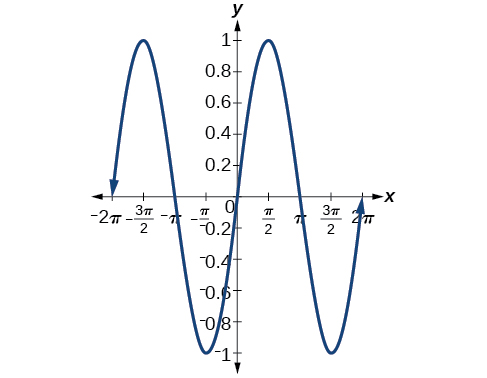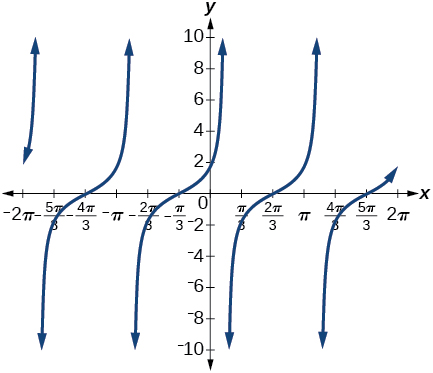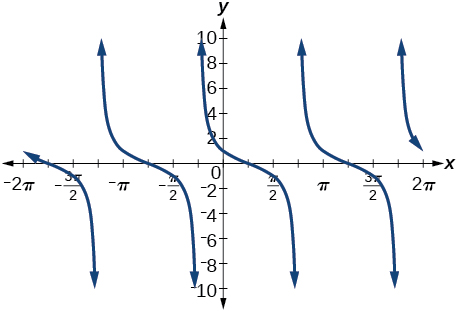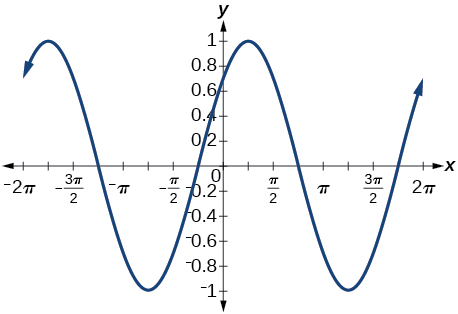# 7.2 Sum and difference identities  (Page 6/6)

 Page 6 / 6

## Verbal

Explain the basis for the cofunction identities and when they apply.

The cofunction identities apply to complementary angles. Viewing the two acute angles of a right triangle, if one of those angles measures $\text{\hspace{0.17em}}x,$ the second angle measures $\text{\hspace{0.17em}}\frac{\pi }{2}-x.\text{\hspace{0.17em}}$ Then $\text{\hspace{0.17em}}\mathrm{sin}x=\mathrm{cos}\left(\frac{\pi }{2}-x\right).\text{\hspace{0.17em}}$ The same holds for the other cofunction identities. The key is that the angles are complementary.

Is there only one way to evaluate $\text{\hspace{0.17em}}\mathrm{cos}\left(\frac{5\pi }{4}\right)?\text{\hspace{0.17em}}$ Explain how to set up the solution in two different ways, and then compute to make sure they give the same answer.

Explain to someone who has forgotten the even-odd properties of sinusoidal functions how the addition and subtraction formulas can determine this characteristic for $\text{\hspace{0.17em}}f\left(x\right)=\mathrm{sin}\left(x\right)\text{\hspace{0.17em}}$ and $\text{\hspace{0.17em}}g\left(x\right)=\mathrm{cos}\left(x\right).\text{\hspace{0.17em}}$ (Hint: $\text{\hspace{0.17em}}0-x=-x$ )

$\mathrm{sin}\left(-x\right)=-\mathrm{sin}x,$ so $\text{\hspace{0.17em}}\mathrm{sin}x\text{\hspace{0.17em}}$ is odd. $\text{\hspace{0.17em}}\mathrm{cos}\left(-x\right)=\mathrm{cos}\left(0-x\right)=\mathrm{cos}x,$ so $\text{\hspace{0.17em}}\mathrm{cos}x\text{\hspace{0.17em}}$ is even.

## Algebraic

For the following exercises, find the exact value.

$\mathrm{cos}\left(\frac{7\pi }{12}\right)$

$\mathrm{cos}\left(\frac{\pi }{12}\right)$

$\frac{\sqrt{2}+\sqrt{6}}{4}$

$\mathrm{sin}\left(\frac{5\pi }{12}\right)$

$\mathrm{sin}\left(\frac{11\pi }{12}\right)$

$\frac{\sqrt{6}-\sqrt{2}}{4}$

$\mathrm{tan}\left(-\frac{\pi }{12}\right)$

$\mathrm{tan}\left(\frac{19\pi }{12}\right)$

$-2-\sqrt{3}$

For the following exercises, rewrite in terms of $\text{\hspace{0.17em}}\mathrm{sin}\text{\hspace{0.17em}}x\text{\hspace{0.17em}}$ and $\text{\hspace{0.17em}}\mathrm{cos}\text{\hspace{0.17em}}x.$

$\mathrm{sin}\left(x+\frac{11\pi }{6}\right)$

$\mathrm{sin}\left(x-\frac{3\pi }{4}\right)$

$-\frac{\sqrt{2}}{2}\mathrm{sin}x-\frac{\sqrt{2}}{2}\mathrm{cos}x$

$\mathrm{cos}\left(x-\frac{5\pi }{6}\right)$

$\mathrm{cos}\left(x+\frac{2\pi }{3}\right)$

$-\frac{1}{2}\mathrm{cos}x-\frac{\sqrt{3}}{2}\mathrm{sin}x$

For the following exercises, simplify the given expression.

$\mathrm{csc}\left(\frac{\pi }{2}-t\right)$

$\mathrm{sec}\left(\frac{\pi }{2}-\theta \right)$

$\mathrm{csc}\theta$

$\mathrm{cot}\left(\frac{\pi }{2}-x\right)$

$\mathrm{tan}\left(\frac{\pi }{2}-x\right)$

$\mathrm{cot}x$

$\mathrm{sin}\left(2x\right)\mathrm{cos}\left(5x\right)-\mathrm{sin}\left(5x\right)\mathrm{cos}\left(2x\right)$

$\frac{\mathrm{tan}\left(\frac{3}{2}x\right)-\mathrm{tan}\left(\frac{7}{5}x\right)}{1+\mathrm{tan}\left(\frac{3}{2}x\right)\mathrm{tan}\left(\frac{7}{5}x\right)}$

$\mathrm{tan}\left(\frac{x}{10}\right)$

For the following exercises, find the requested information.

Given that $\text{\hspace{0.17em}}\mathrm{sin}\text{\hspace{0.17em}}a=\frac{2}{3}\text{\hspace{0.17em}}$ and $\text{\hspace{0.17em}}\mathrm{cos}\text{\hspace{0.17em}}b=-\frac{1}{4},$ with $\text{\hspace{0.17em}}a\text{\hspace{0.17em}}$ and $\text{\hspace{0.17em}}b\text{\hspace{0.17em}}$ both in the interval $\text{\hspace{0.17em}}\left[\frac{\pi }{2},\pi \right),$ find $\text{\hspace{0.17em}}\mathrm{sin}\left(a+b\right)\text{\hspace{0.17em}}$ and $\text{\hspace{0.17em}}\mathrm{cos}\left(a-b\right).$

Given that $\text{\hspace{0.17em}}\mathrm{sin}\text{\hspace{0.17em}}a=\frac{4}{5},$ and $\text{\hspace{0.17em}}\mathrm{cos}\text{\hspace{0.17em}}b=\frac{1}{3},$ with $\text{\hspace{0.17em}}a\text{\hspace{0.17em}}$ and $\text{\hspace{0.17em}}b\text{\hspace{0.17em}}$ both in the interval $\text{\hspace{0.17em}}\left[0,\frac{\pi }{2}\right),$ find $\text{\hspace{0.17em}}\mathrm{sin}\left(a-b\right)\text{\hspace{0.17em}}$ and $\text{\hspace{0.17em}}\mathrm{cos}\left(a+b\right).$

$\mathrm{sin}\left(a-b\right)=\left(\frac{4}{5}\right)\left(\frac{1}{3}\right)-\left(\frac{3}{5}\right)\left(\frac{2\sqrt{2}}{3}\right)=\frac{4-6\sqrt{2}}{15}$
$\mathrm{cos}\left(a+b\right)=\left(\frac{3}{5}\right)\left(\frac{1}{3}\right)-\left(\frac{4}{5}\right)\left(\frac{2\sqrt{2}}{3}\right)=\frac{3-8\sqrt{2}}{15}$

For the following exercises, find the exact value of each expression.

$\mathrm{sin}\left({\mathrm{cos}}^{-1}\left(0\right)-{\mathrm{cos}}^{-1}\left(\frac{1}{2}\right)\right)$

$\mathrm{cos}\left({\mathrm{cos}}^{-1}\left(\frac{\sqrt{2}}{2}\right)+{\mathrm{sin}}^{-1}\left(\frac{\sqrt{3}}{2}\right)\right)$

$\frac{\sqrt{2}-\sqrt{6}}{4}$

$\mathrm{tan}\left({\mathrm{sin}}^{-1}\left(\frac{1}{2}\right)-{\mathrm{cos}}^{-1}\left(\frac{1}{2}\right)\right)$

## Graphical

For the following exercises, simplify the expression, and then graph both expressions as functions to verify the graphs are identical.

$\mathrm{cos}\left(\frac{\pi }{2}-x\right)$

$\mathrm{sin}x$$\mathrm{sin}\left(\pi -x\right)$

$\mathrm{tan}\left(\frac{\pi }{3}+x\right)$

$\mathrm{cot}\left(\frac{\pi }{6}-x\right)$$\mathrm{sin}\left(\frac{\pi }{3}+x\right)$

$\mathrm{tan}\left(\frac{\pi }{4}-x\right)$

$\mathrm{cot}\left(\frac{\pi }{4}+x\right)$$\mathrm{cos}\left(\frac{7\pi }{6}+x\right)$

$\mathrm{sin}\left(\frac{\pi }{4}+x\right)$

$\frac{\mathrm{sin}x}{\sqrt{2}}+\frac{\mathrm{cos}x}{\sqrt{2}}$$\mathrm{cos}\left(\frac{5\pi }{4}+x\right)$

For the following exercises, use a graph to determine whether the functions are the same or different. If they are the same, show why. If they are different, replace the second function with one that is identical to the first. (Hint: think $\text{\hspace{0.17em}}2x=x+x.$ )

$f\left(x\right)=\mathrm{sin}\left(4x\right)-\mathrm{sin}\left(3x\right)\mathrm{cos}\text{\hspace{0.17em}}x,g\left(x\right)=\mathrm{sin}\text{\hspace{0.17em}}x\text{\hspace{0.17em}}\mathrm{cos}\left(3x\right)$

They are the same.

$f\left(x\right)=\mathrm{cos}\left(4x\right)+\mathrm{sin}\text{\hspace{0.17em}}x\text{\hspace{0.17em}}\mathrm{sin}\left(3x\right),g\left(x\right)=-\mathrm{cos}\text{\hspace{0.17em}}x\text{\hspace{0.17em}}\mathrm{cos}\left(3x\right)$

$f\left(x\right)=\mathrm{sin}\left(3x\right)\mathrm{cos}\left(6x\right),g\left(x\right)=-\mathrm{sin}\left(3x\right)\mathrm{cos}\left(6x\right)$

They are the different, try $\text{\hspace{0.17em}}g\left(x\right)=\mathrm{sin}\left(9x\right)-\mathrm{cos}\left(3x\right)\mathrm{sin}\left(6x\right).$

$f\left(x\right)=\mathrm{sin}\left(4x\right),g\left(x\right)=\mathrm{sin}\left(5x\right)\mathrm{cos}\text{\hspace{0.17em}}x-\mathrm{cos}\left(5x\right)\mathrm{sin}\text{\hspace{0.17em}}x$

$f\left(x\right)=\mathrm{sin}\left(2x\right),g\left(x\right)=2\text{\hspace{0.17em}}\mathrm{sin}\text{\hspace{0.17em}}x\text{\hspace{0.17em}}\mathrm{cos}\text{\hspace{0.17em}}x$

They are the same.

$f\left(\theta \right)=\mathrm{cos}\left(2\theta \right),g\left(\theta \right)={\mathrm{cos}}^{2}\theta -{\mathrm{sin}}^{2}\theta$

$f\left(\theta \right)=\mathrm{tan}\left(2\theta \right),g\left(\theta \right)=\frac{\mathrm{tan}\text{\hspace{0.17em}}\theta }{1+{\mathrm{tan}}^{2}\theta }$

They are the different, try $\text{\hspace{0.17em}}g\left(\theta \right)=\frac{2\text{\hspace{0.17em}}\mathrm{tan}\theta }{1-{\mathrm{tan}}^{2}\theta }.$

$f\left(x\right)=\mathrm{sin}\left(3x\right)\mathrm{sin}\text{\hspace{0.17em}}x,g\left(x\right)={\mathrm{sin}}^{2}\left(2x\right){\mathrm{cos}}^{2}x-{\mathrm{cos}}^{2}\left(2x\right){\mathrm{sin}}^{2}x$

$f\left(x\right)=\mathrm{tan}\left(-x\right),g\left(x\right)=\frac{\mathrm{tan}\text{\hspace{0.17em}}x-\mathrm{tan}\left(2x\right)}{1-\mathrm{tan}\text{\hspace{0.17em}}x\text{\hspace{0.17em}}\mathrm{tan}\left(2x\right)}$

They are different, try $\text{\hspace{0.17em}}g\left(x\right)=\frac{\mathrm{tan}x-\mathrm{tan}\left(2x\right)}{1+\mathrm{tan}x\mathrm{tan}\left(2x\right)}.$

## Technology

For the following exercises, find the exact value algebraically, and then confirm the answer with a calculator to the fourth decimal point.

$\mathrm{sin}\left({75}^{\circ }\right)$

$\mathrm{sin}\left({195}^{\circ }\right)$

$\mathrm{cos}\left({165}^{\circ }\right)$

$\mathrm{cos}\left({345}^{\circ }\right)$

$\frac{1+\sqrt{3}}{2\sqrt{2}},$ or 0.9659

$\mathrm{tan}\left(-{15}^{\circ }\right)$

## Extensions

For the following exercises, prove the identities provided.

$\mathrm{tan}\left(x+\frac{\pi }{4}\right)=\frac{\mathrm{tan}\text{\hspace{0.17em}}x+1}{1-\mathrm{tan}\text{\hspace{0.17em}}x}$

$\begin{array}{c}\mathrm{tan}\left(x+\frac{\pi }{4}\right)=\\ \frac{\mathrm{tan}x+\mathrm{tan}\left(\frac{\pi }{4}\right)}{1-\mathrm{tan}x\mathrm{tan}\left(\frac{\pi }{4}\right)}=\\ \frac{\mathrm{tan}x+1}{1-\mathrm{tan}x\left(1\right)}=\frac{\mathrm{tan}x+1}{1-\mathrm{tan}x}\end{array}$

$\frac{\mathrm{tan}\left(a+b\right)}{\mathrm{tan}\left(a-b\right)}=\frac{\mathrm{sin}\text{\hspace{0.17em}}a\text{\hspace{0.17em}}\mathrm{cos}\text{\hspace{0.17em}}a+\mathrm{sin}\text{\hspace{0.17em}}b\text{\hspace{0.17em}}\mathrm{cos}\text{\hspace{0.17em}}b}{\mathrm{sin}\text{\hspace{0.17em}}a\text{\hspace{0.17em}}\mathrm{cos}\text{\hspace{0.17em}}a-\mathrm{sin}\text{\hspace{0.17em}}b\text{\hspace{0.17em}}\mathrm{cos}\text{\hspace{0.17em}}b}$

$\frac{\mathrm{cos}\left(a+b\right)}{\mathrm{cos}\text{\hspace{0.17em}}a\text{\hspace{0.17em}}\mathrm{cos}\text{\hspace{0.17em}}b}=1-\mathrm{tan}\text{\hspace{0.17em}}a\text{\hspace{0.17em}}\mathrm{tan}\text{\hspace{0.17em}}b$

$\begin{array}{c}\frac{\mathrm{cos}\left(a+b\right)}{\mathrm{cos}a\mathrm{cos}b}=\\ \frac{\mathrm{cos}a\mathrm{cos}b}{\mathrm{cos}a\mathrm{cos}b}-\frac{\mathrm{sin}a\mathrm{sin}b}{\mathrm{cos}a\mathrm{cos}b}=1-\mathrm{tan}a\mathrm{tan}b\end{array}$

$\mathrm{cos}\left(x+y\right)\mathrm{cos}\left(x-y\right)={\mathrm{cos}}^{2}x-{\mathrm{sin}}^{2}y$

$\frac{\mathrm{cos}\left(x+h\right)-\mathrm{cos}\text{\hspace{0.17em}}x}{h}=\mathrm{cos}\text{\hspace{0.17em}}x\frac{\mathrm{cos}\text{\hspace{0.17em}}h-1}{h}-\mathrm{sin}\text{\hspace{0.17em}}x\frac{\mathrm{sin}\text{\hspace{0.17em}}h}{h}$

$\begin{array}{c}\frac{\mathrm{cos}\left(x+h\right)-\mathrm{cos}x}{h}=\\ \frac{\mathrm{cos}x\mathrm{cosh}-\mathrm{sin}x\mathrm{sinh}-\mathrm{cos}x}{h}=\\ \frac{\mathrm{cos}x\left(\mathrm{cosh}-1\right)-\mathrm{sin}x\mathrm{sinh}}{h}=\mathrm{cos}x\frac{\mathrm{cos}h-1}{h}-\mathrm{sin}x\frac{\mathrm{sin}h}{h}\end{array}$

For the following exercises, prove or disprove the statements.

$\mathrm{tan}\left(u+v\right)=\frac{\mathrm{tan}\text{\hspace{0.17em}}u+\mathrm{tan}\text{\hspace{0.17em}}v}{1-\mathrm{tan}\text{\hspace{0.17em}}u\text{\hspace{0.17em}}\mathrm{tan}\text{\hspace{0.17em}}v}$

$\mathrm{tan}\left(u-v\right)=\frac{\mathrm{tan}\text{\hspace{0.17em}}u-\mathrm{tan}\text{\hspace{0.17em}}v}{1+\mathrm{tan}\text{\hspace{0.17em}}u\text{\hspace{0.17em}}\mathrm{tan}\text{\hspace{0.17em}}v}$

True

$\frac{\mathrm{tan}\left(x+y\right)}{1+\mathrm{tan}\text{\hspace{0.17em}}x\text{\hspace{0.17em}}\mathrm{tan}\text{\hspace{0.17em}}x}=\frac{\mathrm{tan}\text{\hspace{0.17em}}x+\mathrm{tan}\text{\hspace{0.17em}}y}{1-{\mathrm{tan}}^{2}x\text{\hspace{0.17em}}{\mathrm{tan}}^{2}y}$

If $\text{\hspace{0.17em}}\alpha ,\beta ,$ and $\text{\hspace{0.17em}}\gamma \text{\hspace{0.17em}}$ are angles in the same triangle, then prove or disprove $\text{\hspace{0.17em}}\mathrm{sin}\left(\alpha +\beta \right)=\mathrm{sin}\text{\hspace{0.17em}}\gamma .$

True. Note that $\text{\hspace{0.17em}}\mathrm{sin}\left(\alpha +\beta \right)=\mathrm{sin}\left(\pi -\gamma \right)\text{\hspace{0.17em}}$ and expand the right hand side.

If $\text{\hspace{0.17em}}\alpha ,\beta ,$ and $\text{\hspace{0.17em}}y\text{\hspace{0.17em}}$ are angles in the same triangle, then prove or disprove $\text{\hspace{0.17em}}\mathrm{tan}\text{\hspace{0.17em}}\alpha +\mathrm{tan}\text{\hspace{0.17em}}\beta +\mathrm{tan}\text{\hspace{0.17em}}\gamma =\mathrm{tan}\text{\hspace{0.17em}}\alpha \text{\hspace{0.17em}}\mathrm{tan}\text{\hspace{0.17em}}\beta \text{\hspace{0.17em}}\mathrm{tan}\text{\hspace{0.17em}}\gamma$

#### Questions & Answers

how fast can i understand functions without much difficulty
Joe Reply
what is set?
Kelvin Reply
a colony of bacteria is growing exponentially doubling in size every 100 minutes. how much minutes will it take for the colony of bacteria to triple in size
Divya Reply
I got 300 minutes. is it right?
Patience
no. should be about 150 minutes.
Jason
It should be 158.5 minutes.
Mr
ok, thanks
Patience
100•3=300 300=50•2^x 6=2^x x=log_2(6) =2.5849625 so, 300=50•2^2.5849625 and, so, the # of bacteria will double every (100•2.5849625) = 258.49625 minutes
Thomas
what is the importance knowing the graph of circular functions?
Arabella Reply
can get some help basic precalculus
ismail Reply
What do you need help with?
Andrew
how to convert general to standard form with not perfect trinomial
Camalia Reply
can get some help inverse function
ismail
Rectangle coordinate
Asma Reply
how to find for x
Jhon Reply
it depends on the equation
Robert
yeah, it does. why do we attempt to gain all of them one side or the other?
Melissa
whats a domain
mike Reply
The domain of a function is the set of all input on which the function is defined. For example all real numbers are the Domain of any Polynomial function.
Spiro
Spiro; thanks for putting it out there like that, 😁
Melissa
foci (–7,–17) and (–7,17), the absolute value of the differenceof the distances of any point from the foci is 24.
Churlene Reply
difference between calculus and pre calculus?
Asma Reply
give me an example of a problem so that I can practice answering
Jenefa Reply
x³+y³+z³=42
Robert
dont forget the cube in each variable ;)
Robert
of she solves that, well ... then she has a lot of computational force under her command ....
Walter
what is a function?
CJ Reply
I want to learn about the law of exponent
Quera Reply
explain this
Hinderson Reply

### Read also:

#### Get Jobilize Job Search Mobile App in your pocket Now!

Source:  OpenStax, Precalculus. OpenStax CNX. Jan 19, 2016 Download for free at https://legacy.cnx.org/content/col11667/1.6
Google Play and the Google Play logo are trademarks of Google Inc.

Notification Switch

Would you like to follow the 'Precalculus' conversation and receive update notifications?ByByBy Anindyo MukhopadhyayBy Steve GibbsBy Stephen VoronBy OpenStaxBy Ali SidBy Richley CrapoBy Angela JanuaryBy Cath YuBy Yacoub JayoghliBy Anh Dao